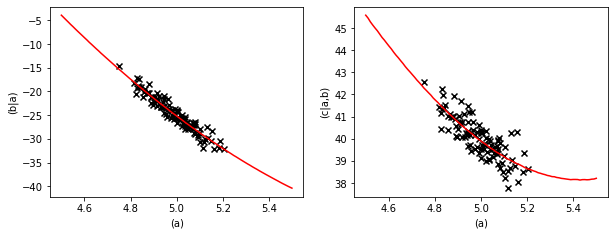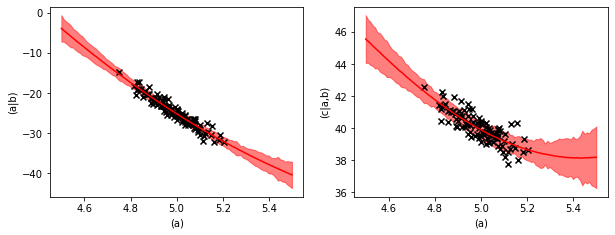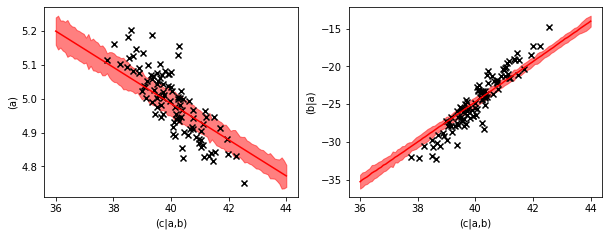# Causal Structures - Prediction#

:

%%capture
# execute the creation & training notebook first
%run "02-01-creation_and_training.ipynb"


In the previous section we created and trained our causal structure. We can now make predictions. Let’s create some test data first.

:

test_data_a = {"(a)": np.linspace(4.5, 5.5, 100)}
prediction = causal_structure.predict(data=test_data_a)


Since we passed a dictionary as data to the predict method, the result is also a dictionary.

:

display(type(prediction))
display(prediction.keys())

pandas.core.frame.DataFrame

Index(['(a)', '(b|a)', '(c|a,b)'], dtype='object')


If we pass a pandas data frame we get a pandas data frame in return.

:

test_data_a = pd.DataFrame(data=test_data_a)
prediction = causal_structure.predict(data=test_data_a)

:

(a) (b|a) (c|a,b)
0 4.500000 -3.950142 45.574075
1 4.510101 -4.436720 45.443720
2 4.520202 -4.927574 45.272895
3 4.530303 -5.408997 45.130555
4 4.540404 -5.907117 44.996075

Note that even though the column ‘(c|a,b)’ depends on both ‘(a)’ and ‘(b|a)’ and we only provided data for ‘(a)’ we get a prediction for ‘(c|a,b)’. This prediction is based on averaging the possible values of ‘(b|a)’ given the provided data for ‘(a)’.

We can plot the prediction and compare it to the training data.

:

pl.figure(figsize=(10,3.5))
fig = pl.subplot(1,2,1)
fig.plot(prediction["(a)"], prediction["(b|a)"], color="red")
fig.scatter(data["(a)"], data["(b|a)"], marker="x", color="k")
fig.set_xlabel("(a)")
fig.set_ylabel("(b|a)")
fig = pl.subplot(1,2,2)
fig.plot(prediction["(a)"], prediction["(c|a,b)"], color="red")
fig.scatter(data["(a)"], data["(c|a,b)"], marker="x", color="k")
fig.set_xlabel("(a)")
fig.set_ylabel("(c|a,b)")

:

Text(0, 0.5, '(c|a,b)')## Prediction uncertainties#

If we want to see the uncertainty margin of the prediction we have to ask the predict method for the standard deviation.

:

prediction_mean, prediction_std = causal_structure.predict(
data=test_data_a, return_std=True)

:

pl.figure(figsize=(10,3.5))
fig = pl.subplot(1,2,1)
fig.plot(prediction_mean["(a)"], prediction_mean["(b|a)"], color="red")
fig.fill_between(prediction_mean["(a)"],
(prediction_mean - prediction_std)["(b|a)"],
(prediction_mean + prediction_std)["(b|a)"],
color="red", alpha=0.5)
fig.scatter(data["(a)"], data["(b|a)"], marker="x", color="k")
fig.set_xlabel("(a)")
fig.set_ylabel("(a|b)")
fig = pl.subplot(1,2,2)
fig.plot(prediction_mean["(a)"], prediction_mean["(c|a,b)"], color="red")
fig.fill_between(prediction_mean["(a)"],
(prediction_mean - prediction_std)["(c|a,b)"],
(prediction_mean + prediction_std)["(c|a,b)"],
color="red", alpha=0.5)
fig.scatter(data["(a)"], data["(c|a,b)"], marker="x", color="k")
fig.set_xlabel("(a)")
fig.set_ylabel("(c|a,b)")

:

Text(0, 0.5, '(c|a,b)')The uncertainty margin consists of two contributions. The learned variance of the quadratic regressions and the uncertainty of the regression parameters.

With the regression equation,

$$y(x) = a \cdot x + b \cdot x^2 + c + \xi$$,

the learned variance describes the strength of the random noise $$\xi$$ and the uncertainty of the regression parameters quantifies that with finite data we know $$a$$, $$b$$, and $$c$$ only with a finite precision.

## Backwards prediction#

We can also evaluate the inverse prediction, e.g. predicting ‘(b|a)’ and ‘(a)’ from ‘(c|a,b)’. We just have to provide data for ‘(c|a,b)’ to the predict method and the causal structure will solve the inverse model.

:

test_data_c = pd.DataFrame(data={'(c|a,b)': np.linspace(36, 44, 100)})

prediction_mean, prediction_std = causal_structure.predict(
data=test_data_c, return_std=True)


:

(a) (b|a) (c|a,b)
0 5.199343 -35.290706 36.000000
1 5.195043 -35.022511 36.080808
2 5.190741 -34.832743 36.161616
3 5.186438 -34.653216 36.242424
4 5.182134 -34.413845 36.323232
:

pl.figure(figsize=(10,3.5))
fig = pl.subplot(1,2,1)
fig.plot(prediction_mean["(c|a,b)"], prediction_mean["(a)"], color="red")
fig.fill_between(prediction_mean["(c|a,b)"],
(prediction_mean - prediction_std)["(a)"],
(prediction_mean + prediction_std)["(a)"],
color="red", alpha=0.5)
fig.scatter(data["(c|a,b)"], data["(a)"], marker="x", color="k")
fig.set_xlabel("(c|a,b)")
fig.set_ylabel("(a)")
fig = pl.subplot(1,2,2)
fig.plot(prediction_mean["(c|a,b)"], prediction_mean["(b|a)"], color="red")
fig.fill_between(prediction_mean["(c|a,b)"],
(prediction_mean - prediction_std)["(b|a)"],
(prediction_mean + prediction_std)["(b|a)"],
color="red", alpha=0.5)
fig.scatter(data["(c|a,b)"], data["(b|a)"], marker="x", color="k")
fig.set_xlabel("(c|a,b)")
fig.set_ylabel("(b|a)")

:

Text(0, 0.5, '(b|a)')The plots of the backwards prediction show us that the backwards prediction usually is a little bit conservative. The predictions stay closer to the average value than the data points.

This is common behavior in inverse modelling and has to do with the fact that a high value of ‘(c|a,b)’ can have multiple causes, a high value in ‘(a)’, a high value in ‘(a|b)’ or a high value of the random noise contribution. This effect is explained in more detail in the inheritance example in the core-documentation.

Apart from predicting values a causal structure can evaluate objectives. We will see what they are in the next section.